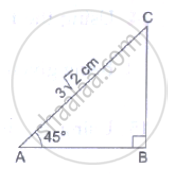# In the Adjoining Figure, δAbc is Right-angled at B and ∠A = 450. If Ac = 3Sqrt(2)Cm, Find (I) Bc, (Ii) Ab. - Mathematics

In the adjoining figure, ΔABC is right-angled at B and ∠A = 450. If AC = 3sqrt(2)cm, find (i) BC, (ii) AB.

#### SolutionFrom the right-angled ΔABC, we have:
(BC)/(AC) = sin 45^0
⇒ (BC)/(3sqrt(2))  = 1/sqrt(2) ⇒ BC = 3cm
Also, (AB)/(AC)  = cos 45^0
⇒ (AB)/(3sqrt(2)) = 1/sqrt(2) ⇒ AB = 3 cm
∴ BC = 3 cm and AB = 3 cm

Concept: Trigonometric Ratios and Its Reciprocal
Is there an error in this question or solution?

#### APPEARS IN

RS Aggarwal Secondary School Class 10 Maths
Chapter 6 T-Ratios of some particular angles
Exercises | Q 22
Share